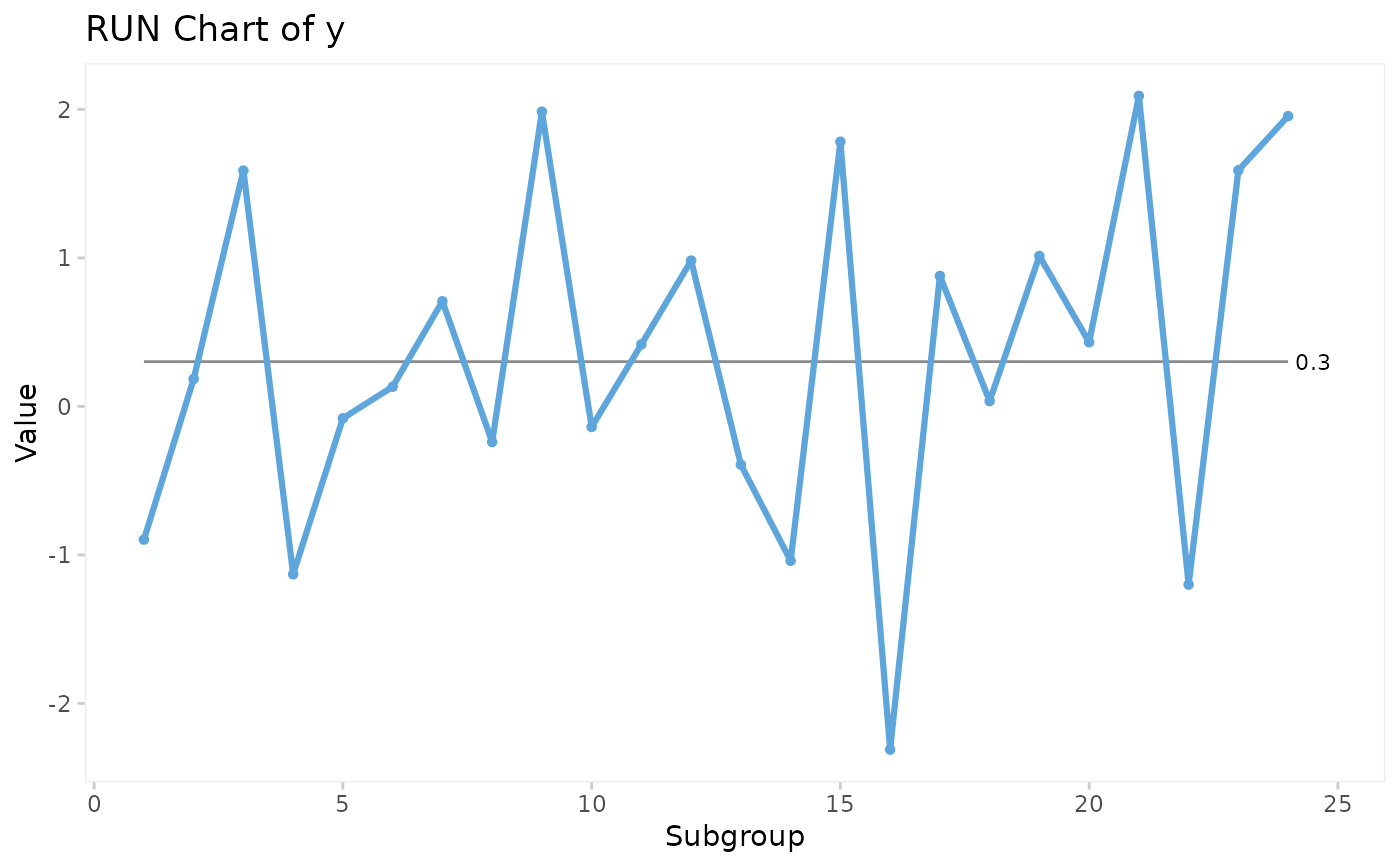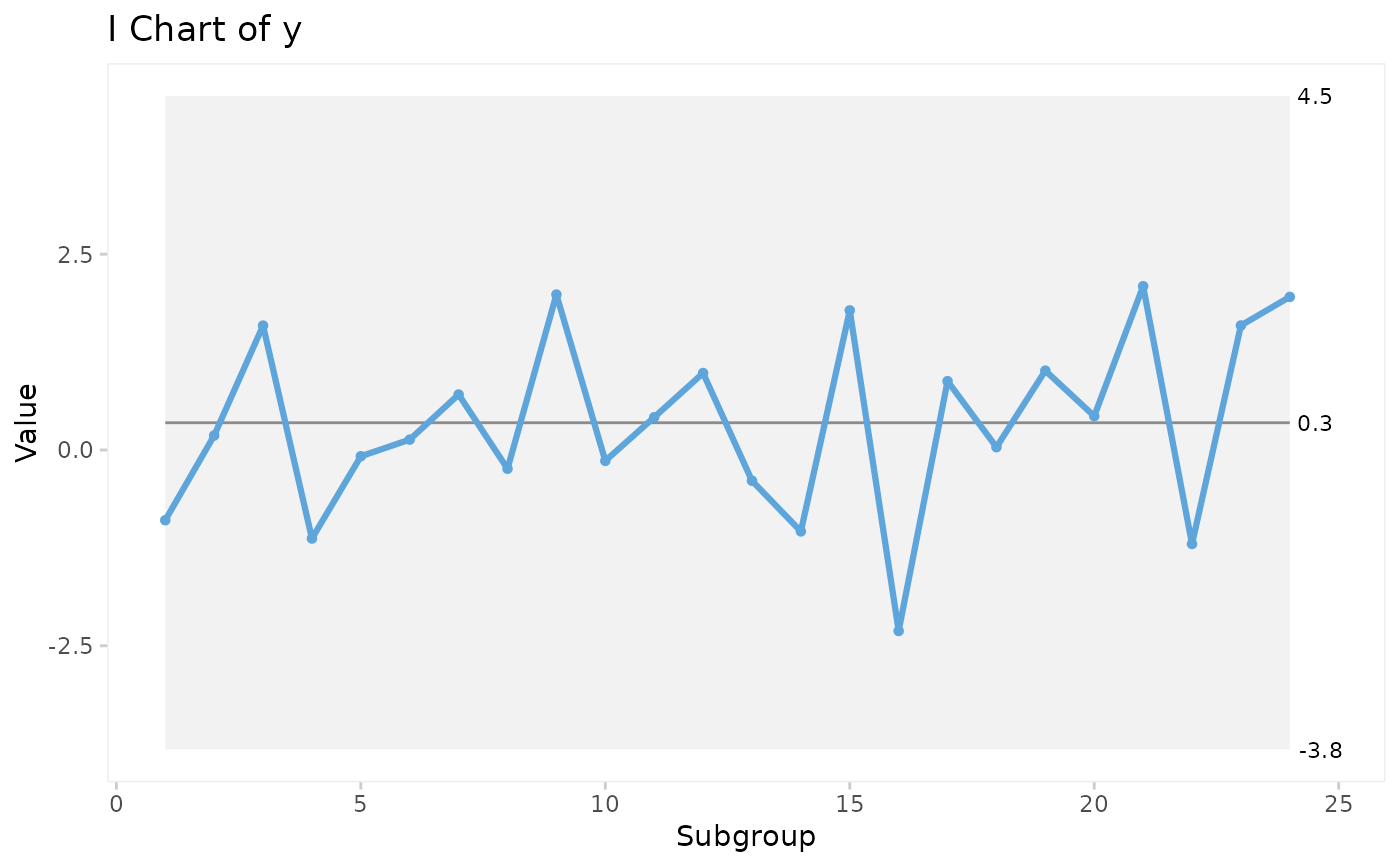The qic() function creates run charts and Shewhart control charts for process control and improvement. Included control charts are: I, MR, Xbar, S, T, C, U, U', P, P', and G charts.

qic(
x,
y = NULL,
n = NULL,
data = NULL,
facets = NULL,
notes = NULL,
chart = c("run", "i", "mr", "xbar", "s", "t", "p", "pp", "c", "u", "up", "g"),
agg.fun = c("mean", "median", "sum", "sd"),
method = c("anhoej", "bestbox", "cutbox"),
multiply = 1,
freeze = NULL,
part = NULL,
exclude = NULL,
target = NA * 1,
cl = NA * 1,
nrow = NULL,
ncol = NULL,
scales = "fixed",
title = "",
ylab = "Value",
xlab = "Subgroup",
subtitle = NULL,
caption = NULL,
part.labels = NULL,
show.labels = is.null(facets),
show.95 = FALSE,
decimals = 1,
point.size = 1.2,
x.period = NULL,
x.format = NULL,
x.angle = NULL,
y.expand = NULL,
y.neg = TRUE,
y.percent = FALSE,
y.percent.accuracy = NULL,
show.grid = FALSE,
flip = FALSE,
strip.horizontal = FALSE,
print.summary = FALSE,
return.data = FALSE
)

## Arguments

x

Vector of subgroup values to plot along the x axis.

y

Vector of measures or counts to plot on the y axis (numerator).

n

Vector of subgroup sizes (denominator).

data

Data frame containing variables used in the plot.

facets

One or two sided formula with factors used for facetting plots.

notes

Character vector of notes to be added to individual data points.

chart

Character value indicating the chart type. Possible values are: 'run' (default), 'i', 'mr', 'xbar', 't', 's', 'c', 'u', 'up', 'p', 'pp', and 'g'.

agg.fun

Aggregate function for summarising the y variable if there are more than one observation per subgroup. Only relevant for run charts and I charts. Possible values are: 'mean' (default), 'median', 'sum', and 'sd'.

method

Character indicating the method used for runs analysis. One of 'anhoej' (default), 'bestbox', and 'cutbox'. EXPERIMENTAL!

multiply

Number indicating a number to multiply y axis by, e.g. 100 for percents rather than proportions. See also y.percent argument.

freeze

Integer indicating the last data point to include in calculation of baseline parameters for centre and control lines. Ignored if part argument is given.

part

Either integer vector indicating data points before recalculation of centre and control lines or character vector indicating chart parts.

exclude

Integer vector indicating data points to exclude from calculations of centre and control lines.

target

Numeric, either a single value indicating a target value to be plotted as a horizontal line or a vector for variable target line.

cl

Numeric, either a single value indicating the centre line if known in advance or a vector for variable centre line.

nrow, ncol

Number indicating the preferred number of rows and columns in facets.

scales

Character string, one of 'fixed' (default), 'free_y', 'free_x', or 'free' indicating whether y and x axis scales should be the same for all panels or free.

title

Character string specifying the title of the plot.

ylab

Character string specifying the y axis label.

xlab

Character string specifying the x axis label.

subtitle

Character string specifying the subtitle.

caption

Character string specifying the caption.

part.labels

Character vector specifying labels for chart parts created with the freeze or part argument.

show.labels

Logical indicating whether to show labels for centre and control lines on chart. Defaults to TRUE when facets argument is NULL.

show.95

Logical indicating whether to show 95 control limits on chart. Defaults to FALSE.

decimals

Integer indicating the preferred number of decimals in centre and control line labels.

point.size

Number specifying the size of data points.

x.period

Character string specifying the interval cut points of datetime x values used for aggregating y values by week, month, etc. See the breaks argument of ?cut.POSIXt() for possible values.

x.format

Date format of x axis labels. See ?strftime() for possible date formats.

x.angle

Number indicating the angle of x axis labels.

Number indicating expansion of x axis to make room for axis labels.

y.expand

Numeric value to include in y axis. Useful e.g. for starting the y axis at zero.

y.neg

If TRUE (default), the y axis is allowed to be negative (only relevant for I and Xbar charts).

y.percent

If TRUE, formats y axis labels as percentages.

y.percent.accuracy

A number to round percentage labels on y axis. Use 1 to show no decimals, 0.1 to show 1 decimal etc.

show.grid

If TRUE, shows grid.

flip

If TRUE, rotates the plot 90 degrees.

strip.horizontal

If TRUE, makes y strip horizontal.

print.summary

If TRUE, prints summary.

return.data

If TRUE, returns underlying data frame.

## Value

A qic object. Inherits from 'ggplot'.

## Details

Non-random variation in the form of minor to moderate persistent shifts in data over time is (by default) identified by the Anhoej rules for unusually long runs and unusually few crossing. Use the EXPERIMENTAL method argument to apply the bestbox or cutbox methods as described in Anhøj & Wentzel-Larsen (2020) doi: 10.1371/journal.pone.0233920 .

Special cause variation in the form of larger, possibly transient, shifts in data is identified by Shewhart's 3-sigma rule (Mohammed, Worthington, Woodall (2008) doi: 10.1136/qshc.2004.012047 ).

vignette('qic').

## Examples

# Lock random number generator to make reproducible results.
set.seed(2)

# Generate vector of 24 random normal numbers
y <- rnorm(24)

# Run chart
qic(y)# I control chart
qic(y, chart = 'i')# U control chart from build-in data set of hospital infection rates faceted
#   by hospital and type of infection.
qic(month, n,
n        = days,
data     = hospital_infections,
facets   = infection ~ hospital,
chart    = 'u',
multiply = 10000,
title    = 'Hospital infection rates',
ylab     = 'Number of infections per 10.000 risk days',
xlab     = 'Month')